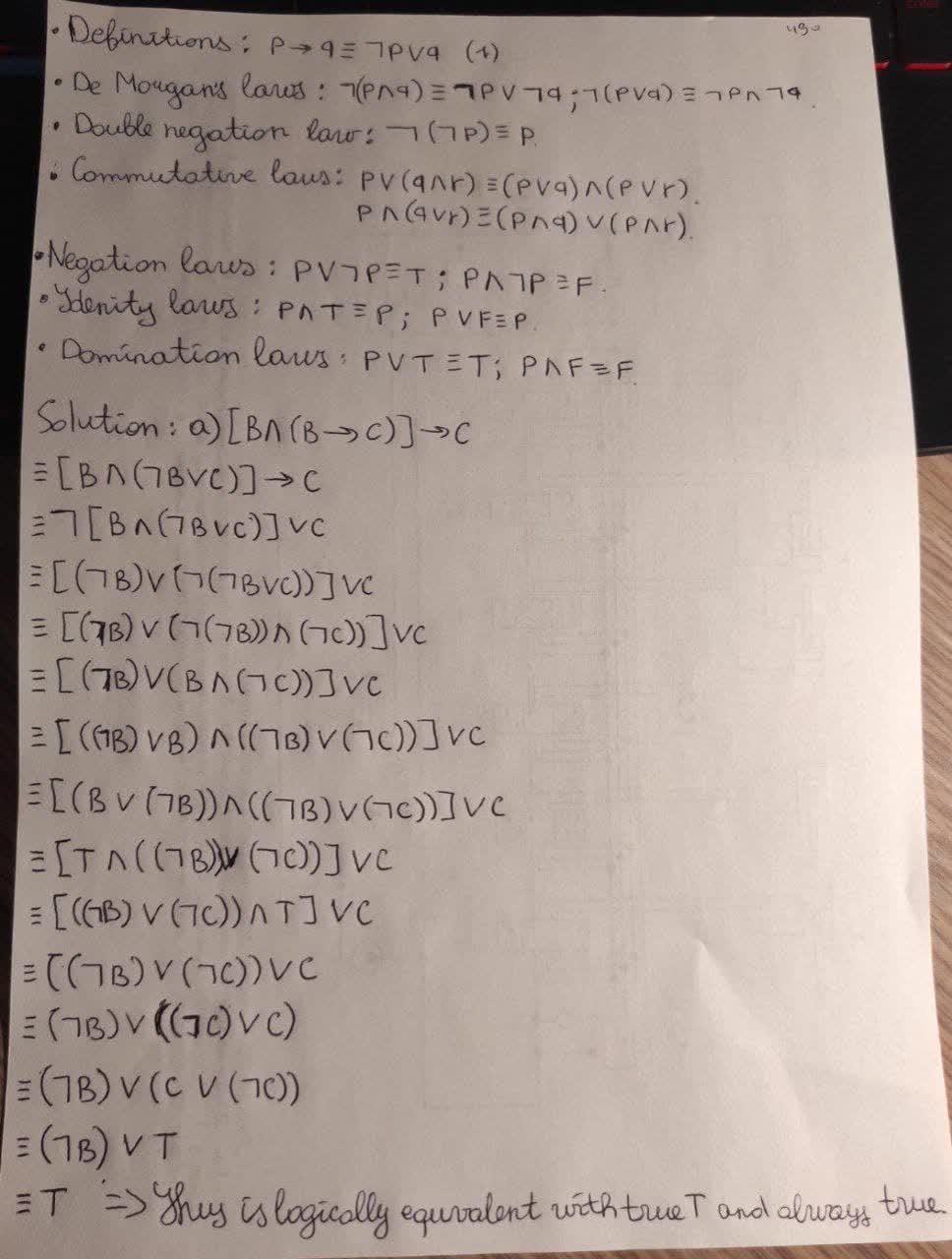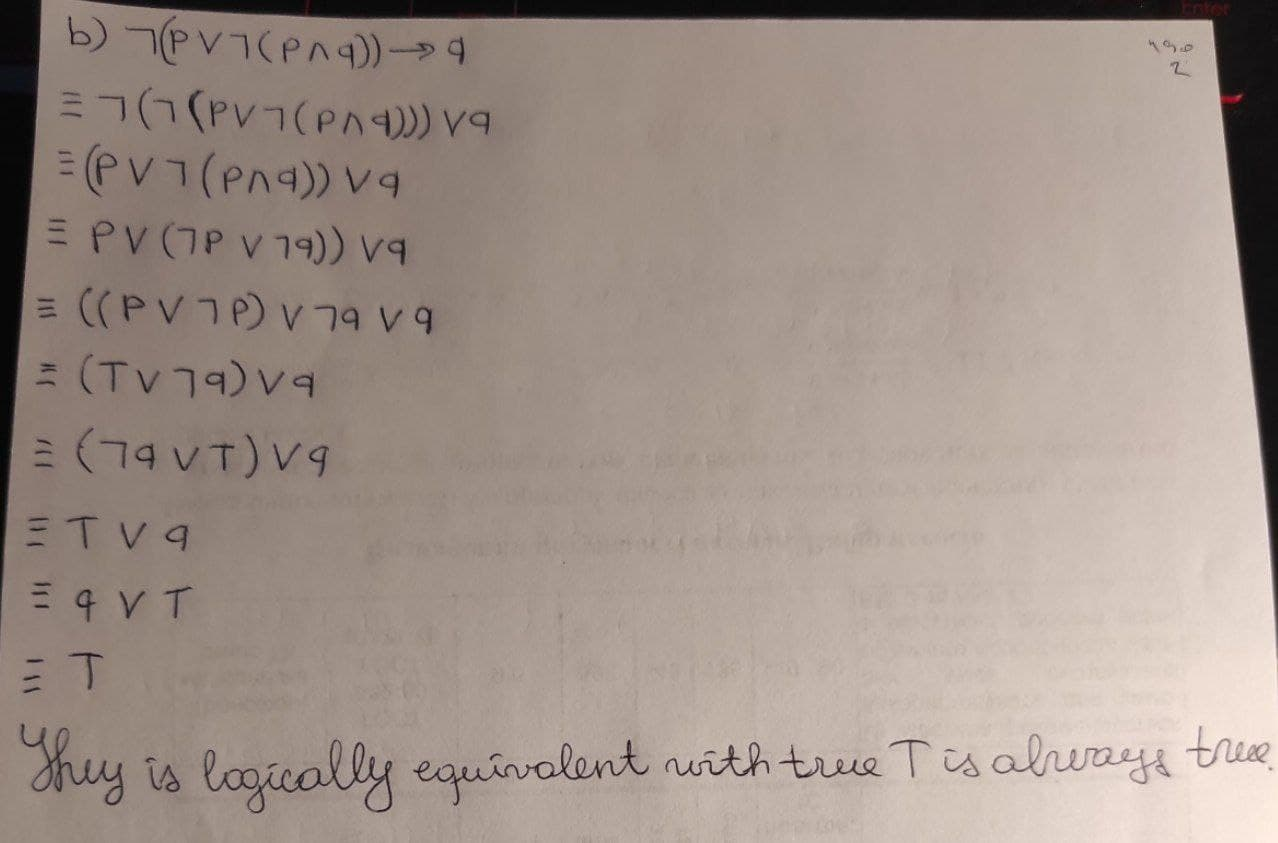# Prove the following relations by Contradiction (Use rules. Don’t use truth table). a) [B ^^ (B rarr C )] rarr C b) not (p vv not (p ∧ q) ) rarr qsmileycellist2 2021-01-08 Answered
Prove the following relations by Contradiction (Use rules. Don’t use truth table).
a) $\left[B\wedge \left(B\to C\right)\right]\to C$
b) $\mathrm{¬}\left(p\vee \mathrm{¬}\left(p\wedge q\right)\right)\to q$
You can still ask an expert for help

## Want to know more about Discrete math?

• Questions are typically answered in as fast as 30 minutes

Solve your problem for the price of one coffee

• Math expert for every subject
• Pay only if we can solve itClelioo